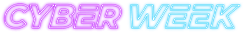#### Save big!

All courses under \$5 - for a limited time!

Code has been added to clipboard!

# Best Way of Using while Loop JavaScript Explained

Published Aug 10, 2017
Updated Oct 2, 2019

One of the key features of JavaScript is a loop: it makes a particular action perform repeatedly, saving you time. All JavaScript loops can be split into two categories: for loops and while loops. JavaScript for loops are again divided into three types: the general for loop, a for/in loop and a for/of loop.

The while loop JavaScript coders use can be of two types: while loop and do/while loop. In this tutorial, you will read about the basic syntax and usage of the JavaScript while loop. We will also explain how it differs from the do/while loop.

## while Loop JavaScript: Main Tips

• The `while` loop executes a block of code as long as the defined condition is true.
• You can also use a do while JavaScript loop. It will execute the code once even if the condition is false.
• You should remember to set the variable to be increased in order to avoid an infinite loop.

## Syntax Rules

When you're writing a while loop JavaScript syntax rules should always be in your mind. It is essential you write it correctly, otherwise you might not be able to achieve the results you want. Take a look at the standard while loop JavaScript syntax:

```while (condition) { code that will be executed }```

In the example below, you can see `(i < 10)` is set as the condition. This means that the code in the loop will run over and over again, as long as the variable `i` is less than 10:

Example
``````while (i < 10) {
example += "Loop counter: " + i;
i++;
}``````

Note: Do not forget to increment the value of the variable to avoid an infinite loop, which will crash your browser.

## do while Loop

The JavaScript`do while` loop is different from `while` loop: using do while loop JavaScript always executes the code at least once - even if the condition is false. Then, it will check the condition, and continue to loop again if it is actually true.

```do { Code that will be executed } while (condition)```

In the syntax snippet above, you can see the main difference from the usual JavaScript while loop: in this case, the condition is defined after the code to be executed. Therefore, it is always run at least once before checking whether the condition is met or not.

In the following example, you can see how in the first loop, the condition is `i < -1`, but the starting count number is `0`, so the condition of the loop is false. The first time the code runs, it will be executed and display `0`, but then it will check the condition and stop from running again.

In the second loop, the starting number is `0`, but the condition is `i < 10`, so the condition is true, and the loop will run and display numbers from `0` to `9`:

Example
``````var text = "";
var i = 0; // the loop starts at 0.

do {
text += "<br>Loop counter " + i;
i++;
} while (i < -1);
document.getElementById("test").innerHTML = text; // will print 0, even though the condition is false.

do {
text += "<br>Loop counter " + i;
i++;
} while (i < 10);
document.getElementById("test2").innerHTML = text; // will print all of the numbers from 0 to 9.``````

## Comparing for to while

`for` and `while` loops are pretty similar, but `while` loop uses only the second one of the `for` loop's three statements.

In this example, `for` loop is used as a `while` loop to get all fruit names from a given array:

Example
``````var fruits = ["Banana", "Orange", "Cherry", "Apple"];
var i = 0;
var text = "";
for (;fruits[i];) {
text += fruits[i] + "<br>";
i++;
}``````

Now, in this example, `while` loop is used to get all fruit names from a given array.

Example
``````var fruits = ["Apple", "Banana", "Cherry", "Orange"];
var i = 0;
var example = "";
while (fruits[i]) {
example += fruits[i] + "<br>";
i++;
}``````

Don't hesitate to push Try it Live buttons and see the differences for yourself. This way, you will understand the material much quicker, and be able to get a better idea on how to use it yourself.

## while Loop JavaScript: Summary

• There are two types of `while` loops: `while` and `do/while`.
• Using while loop JavaScript runs a block of code if the condition is true.
• JavaScript do while loop runs at least once, even if the condition is false.